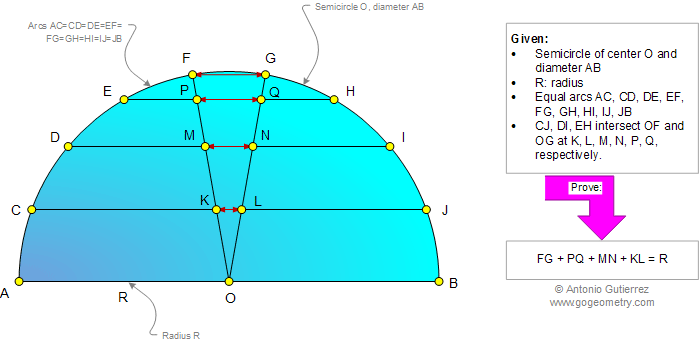# Online Geometry Problem 927: Semicircle, Diameter, Radius, Equal Arcs, Chord, Congruence. Level: High School, SAT Prep, College, Mathematics Education

< PREVIOUS PROBLEM  |  NEXT PROBLEM >

 The figure below shows a semicircle of center O, diameter AB, radius R and equal arcs AC, CD, DE, EF, FG, GH, HI, IJ, JB. Chords CJ, DI, and EH intersect OF and OG at K, L, M, N, P, Q, respectively. Prove that FG + PQ + MN + KL = R. Try to use elementary geometry (Euclid's Elements.)Home | Search | Geometry | Problems | All Problems | Open Problems | Visual Index | 10 Problems | 921-930 | Triangles | Circles | Semicircle | Congruence | Email | Solution / comment | By Antonio Gutierrez